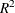The SYSLIN Procedure

SUR, 3SLS, and FIML Estimation

Subsections:

In a multivariate regression model, the errors in different equations might be correlated. In this case, the efficiency of the estimation might be improved by taking these cross-equation correlations into account.

Seemingly Unrelated Regression

Seemingly unrelated regression (SUR), also called joint generalized least squares (JGLS) or Zellner estimation, is a generalization of OLS for multi-equation systems. Like OLS, the SUR method assumes that all the regressors are independent variables, but SUR uses the correlations among the errors in different equations to improve the regression estimates. The SUR method requires an initial OLS regression to compute residuals. The OLS residuals are used to estimate the cross-equation covariance matrix.

The SUR option in the PROC SYSLIN statement specifies seemingly unrelated regression, as shown in the following statements:

```proc syslin data=in sur;
demand: model q = p y s;
supply: model q = p u;
run;
```

INSTRUMENTS and ENDOGENOUS statements are not needed for SUR, because the SUR method assumes there are no endogenous regressors. For SUR to be effective, the models must use different regressors. SUR produces the same results as OLS unless the model contains at least one regressor not used in the other equations.

Three-Stage Least Squares

The three-stage least squares method generalizes the two-stage least squares method to take into account the correlations between equations in the same way that SUR generalizes OLS. Three-stage least squares requires three steps: first-stage regressions to get predicted values for the endogenous regressors; a two-stage least squares step to get residuals to estimate the cross-equation correlation matrix; and the final 3SLS estimation step.

The 3SLS option in the PROC SYSLIN statement specifies the three-stage least squares method, as shown in the following statements.

```proc syslin data=in 3sls;
endogenous  p;
instruments y u s;
demand: model q = p y s;
supply: model q = p u;
run;
```

The 3SLS output begins with a two-stage least squares regression to estimate the cross-model correlation matrix. This output is the same as the 2SLS results shown in Figure 29.3 and Figure 29.4, and is not repeated here. The next part of the 3SLS output prints the cross-model correlation matrix computed from the 2SLS residuals. This output is shown in Figure 29.5 and includes the cross-model covariances, correlations, the inverse of the correlation matrix, and the inverse covariance matrix.

Figure 29.5: Estimated Cross-Model Covariances Used for 3SLS Estimates

The SYSLIN Procedure
Three-Stage Least Squares Estimation

Cross Model Covariance
DEMAND SUPPLY
DEMAND 0.027892 -.011283
SUPPLY -.011283 0.018991

Cross Model Correlation
DEMAND SUPPLY
DEMAND 1.00000 -0.49022
SUPPLY -0.49022 1.00000

Cross Model Inverse Correlation
DEMAND SUPPLY
DEMAND 1.31634 0.64530
SUPPLY 0.64530 1.31634

Cross Model Inverse Covariance
DEMAND SUPPLY
DEMAND 47.1941 28.0379
SUPPLY 28.0379 69.3130

The final 3SLS estimates are shown in Figure 29.6.

Figure 29.6: Three-Stage Least Squares Results

 System Weighted MSE 0.5711 113 0.9627

Model DEMAND q Quantity

Parameter Estimates
Variable DF Parameter
Estimate
Standard
Error
t Value Pr > |t| Variable
Label
Intercept 1 1.980269 1.169176 1.69 0.0959 Intercept
p 1 -1.17654 0.605015 -1.94 0.0568 Price
y 1 0.404117 0.117179 3.45 0.0011 Income
s 1 0.359204 0.085077 4.22 <.0001 Price of Substitutes

Model SUPPLY q Quantity

Parameter Estimates
Variable DF Parameter
Estimate
Standard
Error
t Value Pr > |t| Variable
Label
Intercept 1 -0.51878 0.490999 -1.06 0.2952 Intercept
p 1 1.333080 0.059271 22.49 <.0001 Price
u 1 -1.14623 0.243491 -4.71 <.0001 Unit Cost

This output first prints the system weighted mean squared error and system weightedstatistics. The system weighted MSE and system weightedmeasure the fit of the joint model obtained by stacking all the models together and performing a single regression with the stacked observations weighted by the inverse of the model error variances. See the section The R-Square Statistics for details.

Next, the table of 3SLS parameter estimates for each model is printed. This output has the same form as for the other estimation methods.

Note that, in some cases, the 3SLS and 2SLS results can be the same. Such a case could arise because of the same principle that causes OLS and SUR results to be identical, unless an equation includes a regressor not used in the other equations of the system. However, the application of this principle is more complex when instrumental variables are used. When all the exogenous variables are used as instruments, linear combinations of all the exogenous variables appear in the third-stage regressions through substitution of first-stage predicted values.

In this example, 3SLS produces different (and, it is hoped, more efficient) estimates for the demand equation. However, the 3SLS and 2SLS results for the supply equation are the same. This is because the supply equation has one endogenous regressor and one exogenous regressor not used in other equations. In contrast, the demand equation has fewer endogenous regressors than exogenous regressors not used in other equations in the system.

Full Information Maximum Likelihood

The FIML option in the PROC SYSLIN statement specifies the full information maximum likelihood method, as shown in the following statements.

```proc syslin data=in fiml;
endogenous  p q;
instruments y u s;
demand: model q = p y s;
supply: model q = p u;
run;
```

The FIML results are shown in Figure 29.7.

Figure 29.7: FIML Results

The SYSLIN Procedure
Full-Information Maximum Likelihood Estimation

 NOTE: Convergence criterion met at iteration 3.

Model DEMAND q Quantity

Parameter Estimates
Variable DF Parameter
Estimate
Standard
Error
t Value Pr > |t| Variable
Label
Intercept 1 1.988538 1.233632 1.61 0.1126 Intercept
p 1 -1.18148 0.652278 -1.81 0.0755 Price
y 1 0.402312 0.107270 3.75 0.0004 Income
s 1 0.361345 0.103817 3.48 0.0010 Price of Substitutes

Model SUPPLY q Quantity

Parameter Estimates
Variable DF Parameter
Estimate
Standard
Error
t Value Pr > |t| Variable
Label
Intercept 1 -0.52443 0.479522 -1.09 0.2787 Intercept
p 1 1.336083 0.057939 23.06 <.0001 Price
u 1 -1.14804 0.237793 -4.83 <.0001 Unit Cost